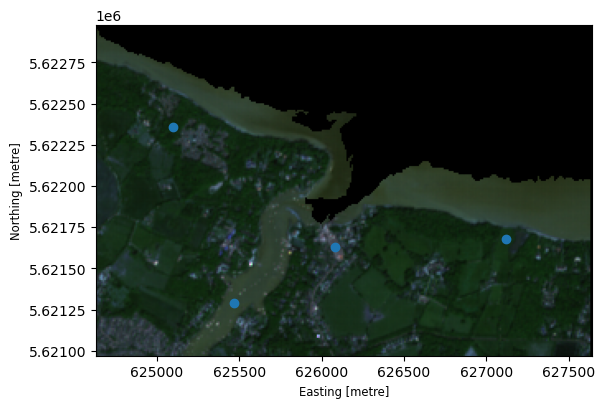# Using GeoPandas with Rasterio to sample point data#

This example shows how to use GeoPandas with Rasterio. Rasterio is a package for reading and writing raster data.

In this example a set of vector points is used to sample raster data at those points.

The raster data used is Copernicus Sentinel data 2018 for Sentinel data.

:

import geopandas
import rasterio
import matplotlib.pyplot as plt
from shapely.geometry import Point


# Create example vector data#

Generate a geodataframe from a set of points

:

# Create sampling points
points = [
Point(625466, 5621289),
Point(626082, 5621627),
Point(627116, 5621680),
Point(625095, 5622358),
]
gdf = geopandas.GeoDataFrame([1, 2, 3, 4], geometry=points, crs=32630)


The GeoDataFrame looks like this:

:

gdf.head()

:

0 geometry
0 1 POINT (625466.000 5621289.000)
1 2 POINT (626082.000 5621627.000)
2 3 POINT (627116.000 5621680.000)
3 4 POINT (625095.000 5622358.000)

# Open the raster data#

Use rasterio to open the raster data to be sampled

:

src = rasterio.open("s2a_l2a_fishbourne.tif")


Let’s see the raster data with the point data overlaid.

:

from rasterio.plot import show

fig, ax = plt.subplots()

# transform rasterio plot to real world coords
extent = [src.bounds, src.bounds, src.bounds, src.bounds]
ax = rasterio.plot.show(src, extent=extent, ax=ax, cmap="pink")

gdf.plot(ax=ax)

:

<Axes: ># Sampling the data#

Rasterio requires a list of the coordinates in x,y format rather than as the points that are in the geomentry column.

This can be achieved using the code below

:

coord_list = [(x, y) for x, y in zip(gdf["geometry"].x, gdf["geometry"].y)]


Carry out the sampling of the data and store the results in a new column called value. Note that if the image has more than one band, a value is returned for each band.

:

gdf["value"] = [x for x in src.sample(coord_list)]

: